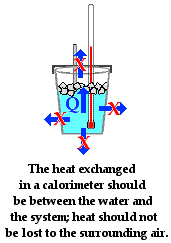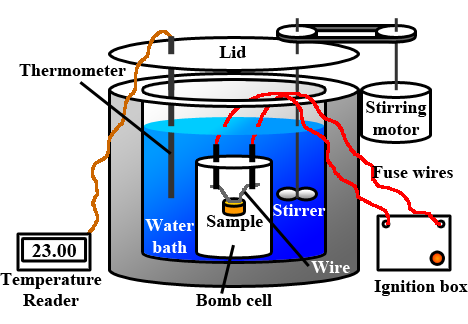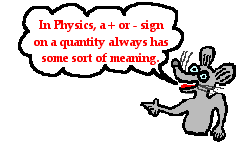Thermal Physics - Lesson 2 Calorimetry

# Calorimeters and Calorimetry

Calorimetry is the science associated with determining the changes in energy of a system by measuring the heat exchanged with the surroundings. Now that sounds very textbooky; but in this last part of Lesson 2, we are going to try to make some meaning of this definition of calorimetry. In physics class (and for some, in chemistry class), calorimetry labs are frequently performed in order to determine the heat of reaction or the heat of fusion or the heat of dissolution or even the specific heat capacity of a metal. These types of labs are rather popular because the equipment is relatively inexpensive and the measurements are usually straightforward. In such labs, a calorimeter is used. A calorimeter is a device used to measure the quantity of heat transferred to or from an object. Most students likely do not remember using such a fancy piece of equipment known as a calorimeter. Fear not; the reason for the lack of memory is not a sign of early Alzheimer's. Rather, it is because the calorimeter used in high school science labs is more commonly referred to as a Styrofoam cup. It is a coffee cup calorimeter - usually filled with water. The more sophisticated cases include a lid on the cup with an inserted thermometer and maybe even a stirrer.

### Coffee Cup Calorimetry

So how can such simple equipment be used to measure the quantity of heat gained or lost by a system? We have learned on the previous page, that water will change its temperature when it gains or loses energy. And in fact, the quantity of energy gained or lost is given by the equation

Q = mwater•Cwater•ΔTwater

where Cwater is 4.18 J/g/°C. So if the mass of water and the temperature change of the water in the coffee cup calorimeter can be measured, the quantity of energy gained or lost by the water can be calculated.The assumption behind the science of calorimetry is that the energy gained or lost by the water is equal to the energy lost or gained by the object under study. So if an attempt is being made to determine the specific heat of fusion of ice using a coffee cup calorimeter, then the assumption is that the energy gained by the ice when melting is equal to the energy lost by the surrounding water. It is assumed that there is a heat exchange between the iceand the water in the cup and that no other objects are involved in the heat exchanged. This statement could be placed in equation form as

Qice = - Qsurroundings = -Qcalorimeter

The role of the Styrofoam in a coffee cup calorimeter is that it reduces the amount of heat exchange between the water in the coffee cup and the surrounding air. The value of a lid on the coffee cup is that it also reduces the amount of heat exchange between the water and the surrounding air. The more that these other heat exchanges are reduced, the more true that the above mathematical equation will be. Any error analysis of a calorimetry experiment must take into consideration the flow of heat from system to calorimeter to other parts of the surroundings. And any design of a calorimeter experiment must give attention to reducing the exchanges of heat between the calorimeter contents and the surroundings.

### Bomb Calorimetry

The coffee cup calorimeters used in high school science labs provides students with a worthwhile exercise in calorimetry. But at the professional level, a cheap Styrofoam cup and a thermometer isn't going to assist a commercial food manufacturer in determining the Calorie content of their products. For situations in which exactness and accuracy is at stake, a more expensive calorimeter is needed. Chemists often use a device known as a bomb calorimeter to measure the heat exchanges associated with chemical reactions, especially combustion reactions. Having little to nothing to do with bombs of the military variety, a bomb calorimeter includes a reaction chamber where the reaction (usually a combustion reaction) takes place. The reaction chamber is a strong vessel that can withstand the intense pressure of heated gases with exploding. The chamber is typically filled with mostly oxygen gas and the fuel. An electrical circuit is wired into the chamber in order to electrically ignite the contents in order to perform a study of the heat released upon combustion. The reaction chamber is surrounded by a jacket of water with a thermometer inserted. The heat released from the chamber warms the water-filled jacket, allowing a scientist to determine the quantity of energy released by the reaction.Source: Wikimedia Commons; thanks to Lisdavid89.

### Solving Calorimetry Problems

Now let's look at a few examples of how a coffee cup calorimeter can be used as a tool to answer some typical lab questions. The next three examples are all based on laboratory experiments involving calorimetry.

Example Problem 1:
A physics class has been assigned the task of determining an experimental value for the heat of fusion of ice. Anna Litical and Noah Formula dry and mass out 25.8-gram of ice and place it into a coffee cup with 100.0 g of water at 35.4°C. They place a lid on the coffee cup and insert a thermometer. After several minutes, the ice has completely melted and the water temperature has lowered to 18.1°C. What is their experimental value for the specific heat of fusion of ice?

The basis for the solution to this problem is the recognition that the quantity of energy lost by the water when cooling is equal to the quantity of energy required to melt the ice. In equation form, this could be stated as

Qice = -Qcalorimeter

(The negative sign indicates that the ice is gaining energy and the water in the calorimeter is losing energy.) Here the calorimeter (as in the Qcalorimeter term) is considered to be the water in the coffee cup. Since the mass of this water and its temperature change are known, the value of Qcalorimeter can be determined.

Qcalorimeter = m•C•ΔT
Qcalorimeter = (100.0 g)•(4.18 J/g/°C)•(18.1°C - 35.4°C)
Qcalorimeter = -7231.4 JThe negative sign indicates that the water lost energy. The assumption is that this energy lost by the water is equal to the quantity of energy gained by the ice. So Qice = +7231.4 J. (The positive sign indicates an energy gain.) This value can be used with the equation from the previous page to determine the heat of fusion of the ice.

Qice = mice•ΔHfusion-ice
+7231.4 J = (25.8 g)•ΔHfusion-ice
ΔHfusion-ice = (+7231.4 J)/(25.8 g)
ΔHfusion-ice = 280.28 J/g
ΔHfusion-ice = 2.80x102 J/g (rounded to two significant figures)

Example Problem 2:
A chemistry student dissolves 4.51 grams of sodium hydroxide in 100.0 mL of water at 19.5°C (in a calorimeter cup). As the sodium hydroxide dissolves, the temperature of the surrounding water increases to 31.7°C. Determine the heat of solution of the sodium hydroxide in J/g.

Once more, the solution to this problem is based on the recognition that the quantity of energy released when sodium hydroxide dissolves is equal to the quantity of energy absorbed by the water in the calorimeter. In equation form, this could be stated asQNaOH dissolving = -Qcalorimeter

(The negative sign indicates that the NaOH is losing energy and the water in the calorimeter is gaining energy.) Since the mass and temperature change of the water have been measured, the energy gained by the water (calorimeter) can be determined.

Qcalorimeter = m•C•ΔT
Qcalorimeter = (100.0 g)•(4.18 J/g/°C)•(31.7°C - 19.5°C)
Qcalorimeter = 5099.6 J

The assumption is that this energy gained by the water is equal to the quantity of energy released by the sodium hydroxide when dissolving. So QNaOH-dissolving = -5099.6 J. (The negative sign indicates an energy lost.) This quantity is the amount of heat released when dissolving 4.51 grams of the sodium hydroxide. When the heat of solution is determined on a per gram basis, this 5099.6 J of energy must be divided by the mass of sodium hydroxide that is being dissolved.

ΔHsolution = QNaOH-dissolving / mNaOH
ΔHsolution = (-5099.6 J) / (4.51 g)
ΔHsolution = -1130.7 J/g
ΔHsolution = -1.13x103 J/g (rounded to three significant figures)

Example Problem 3:
A large paraffin candle has a mass of 96.83 gram. A metal cup with 100.0 mL of water at 16.2°C absorbs the heat from the burning candle and increases its temperature to 35.7°C. Once the burning is ceased, the temperature of the water was 35.7°C and the paraffin had a mass of 96.14 gram. Determine the heat of combustion of paraffin in kJ/gram. GIVEN: density of water = 1.0 g/mL.

As is always the case, calorimetry is based on the assumption that all the heat lost by the system is gained by the surroundings. It is assumed that the surroundings is the water that undergoes the temperature change. In equation form, it could be stated that

Qparaffin = -Qwater

Since the mass and temperature change of the water are known, the energy gained by the water in the calorimeter can be determined.

Qcalorimeter = m•C•ΔT
Qcalorimeter = (100.0 g)•(4.18 J/g/°C)•(35.7°C - 16.2°C)
Qcalorimeter = 8151 J

The paraffin released 8151 J or 8.151 kJ of energy when burned. This is based on the burning of 0.69 gram (96.83 g - 96.14 g). To determine the heat of combustion on a per gram basis, the Qparaffin value (-8.151 kJ) must be divided by the mass of paraffin burned:

ΔHcombustion - paraffin = (-8.151 kJ) / (0.69 g)
ΔHcombustion - paraffin = -11.813 kJ/g
ΔHcombustion - paraffin = -12 kJ/g (rounded to two significant digits)

1. Consider Example Problem 3 above. Identify as many sources of error as you can. For each source indicate the direction of error that would have resulted. That is, identify whether the error would have caused the experimentally derived value to be less than or more than the accepted value.

2. A 2.15-gram cashew nut is burned. The heat released raises the temperature of a 100.0-gram sample of water from 18.2°C to 31.5°C. The mass of the nut after the experiment is 1.78 grams. Determine the calorie content of the nut in Calories/gram. Assume that the water is only able to absorb 25% of the heat released by the burning nut. Given 1.00 Calorie=4.18 kJ.